Categories

# FMSP Favourite Problems – Number 3

After far too long an hiatus I am continuing with my discussion posts on the 6 problems that the Further Mathematics Support Programme, FMSP (@furthermaths) have selected for their “Favourite Problems” poster series.

Problem 3 is described on their poster shown below: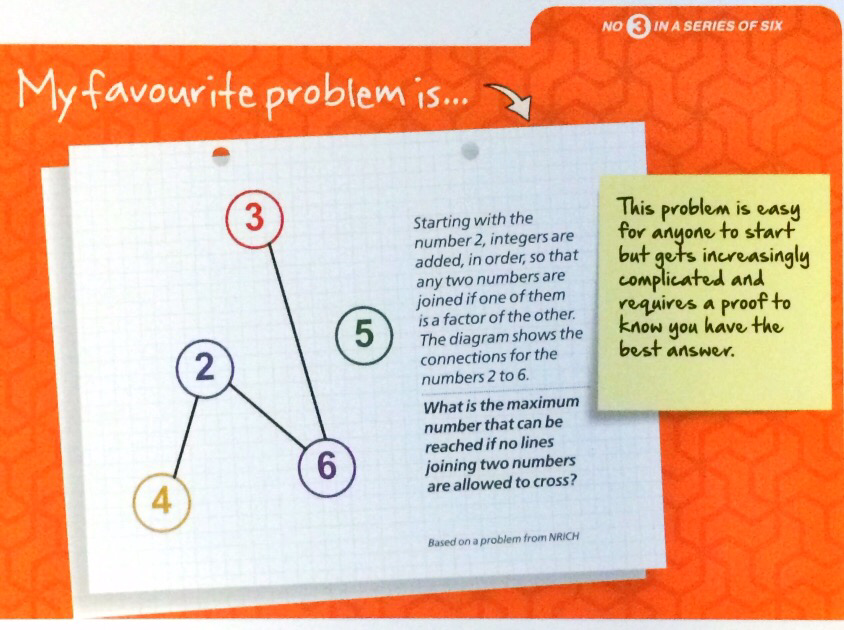This is actually a pretty interesting problem, with a very low threshold for pupils to attempt – as long as they can generate a table of factors of a number they can make a start by adding numbers and connections. For example , we have the next 5 numbers:

• 8 needs to be connected to 2 and 4.
• 9 needs to be connected to 3.
• 10 needs to be connected to 2 and 5.

Adding these numbers gives the following diagram: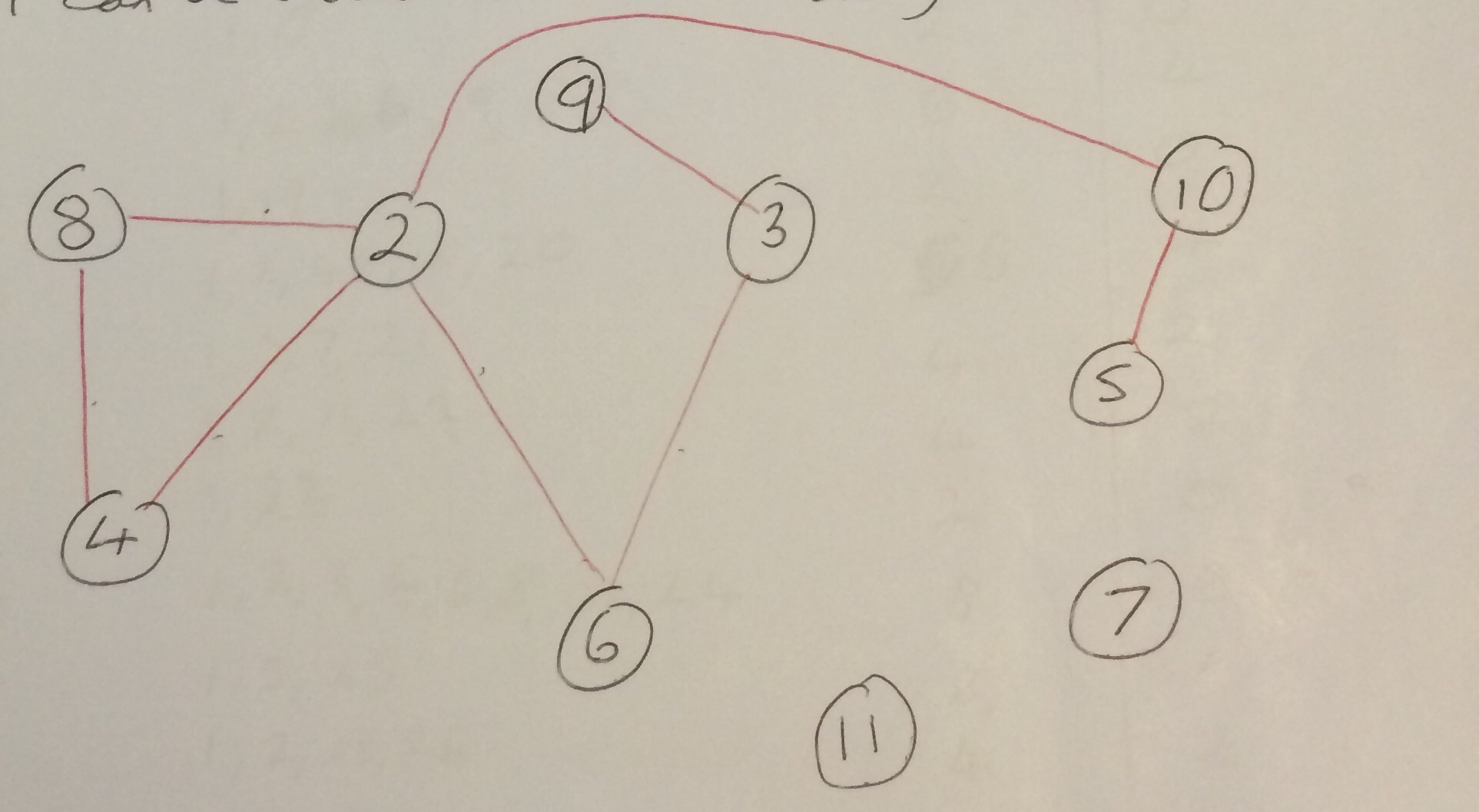If we consider the integers as the vertices of a graph, then this problem becomes that of finding the smallest number such that we cannot draw a planar graph where the vertices are connected in the way described above. A graph is planar if none of the edges cross each other.

Fairly quickly, you realise that the numbers that will be hard to add to the diagram are those with lots of factors, so my next step was to draw up a table looking at the number of factors (excluding 1 and itself) a number has

[table id=factors /]

Looking at this table 24 and 30 stand out as they have 6 factors, the most of the numbers in the table. So lets look at the smallest of the two, 24. 24 has the following 6 factors when you exclude 1 and 24: 2, 3, 4, 6, 8, 12.

To show that it is not possible to draw the diagram for 24 we need to introduce the concept of a complete graph. A complete graph is one such that any pair of vertices of the graph is connected by a unique edge, or to put it another way, every vertex is joined to every other vertex by an edge. It is standard to denote the complete graph with $$n$$ vertices as $$K_n$$. Now it is known that $$K_4$$is planar and $$K_5$$ is non-planar: To see this we can use the planarity testing algorithm studied at A-Level (see below) on $$K_5$$ as shown below.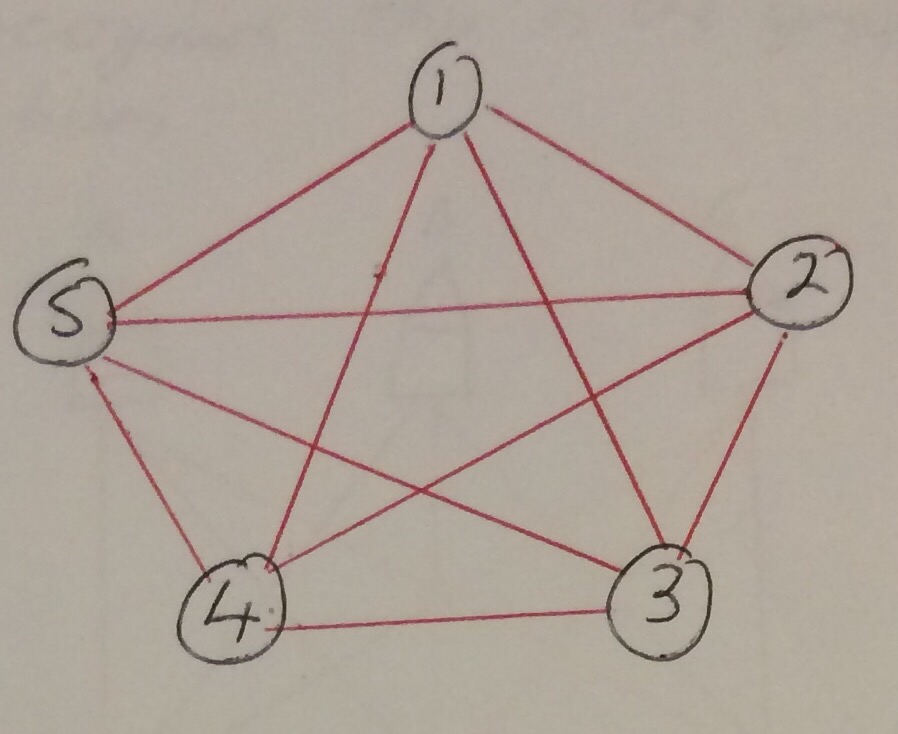1-2-3-4-5 form a Hamiltonian cycle, and we can then move edges 2-5 and 2-4 outside without introducing an edge crossing. However no other edges can be moved outside without introducing a crossing and so $$K_5$$ is non-planar.In fact whether any graph is planar can be related to $$K_5$$ by Kuratowski’s theorem, which was first published by the Polish mathematician Kazimierz Kuratowski in 1930. This well known theorem in graph theory states that “graph is planar if and only if it does not contain a subgraph that is homeomorphic to $$K_5$$ or $$K_{3,3}$$“. In fact the theorem is slightly more general to this, see Wikipedia for a short introduction.

So, if we can show that constructing the graph to include all integers from 2 to 24 would contain a $$K_5$$ graph then we can say that the graph is necessarily non-planar.

Taking 4 of the factors of 24, namely 2,3,6 and 12 and we can draw the following graph:These numbers have been chosen so that 24,2,6 and 12 form the complete graph $$K_4$$ so that it is planar (i.e. there are no crossing lines), as shown below.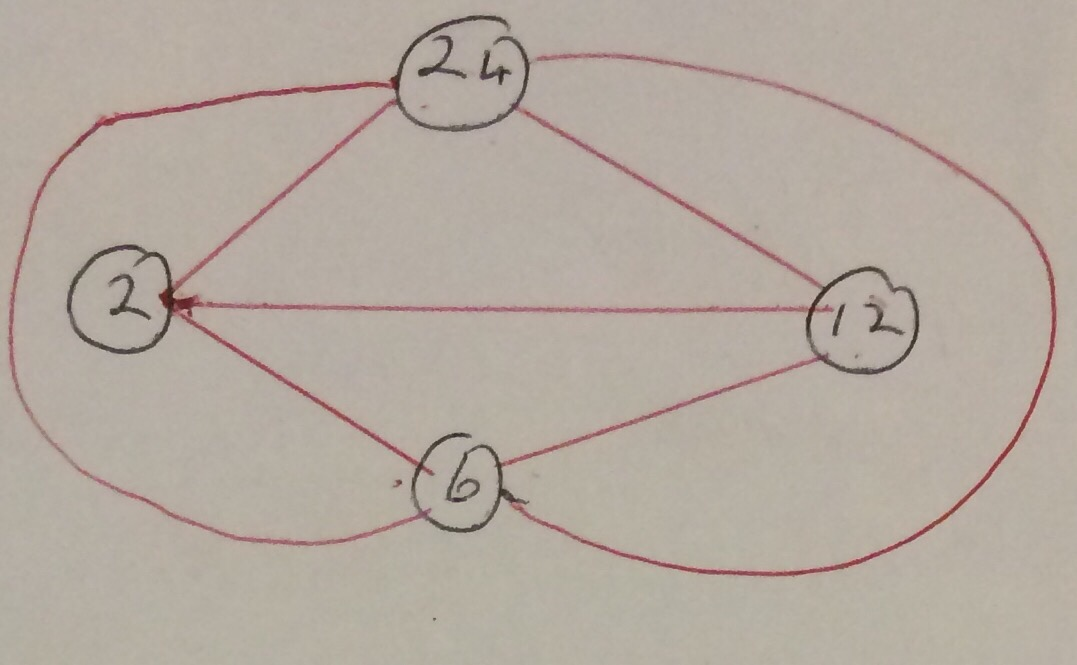Can we add another factor – let’s try adding 3. 3 of course joins to 12, 24 and 6, but it doesn’t connect to 3, so at the moment we don’t have a complete graph.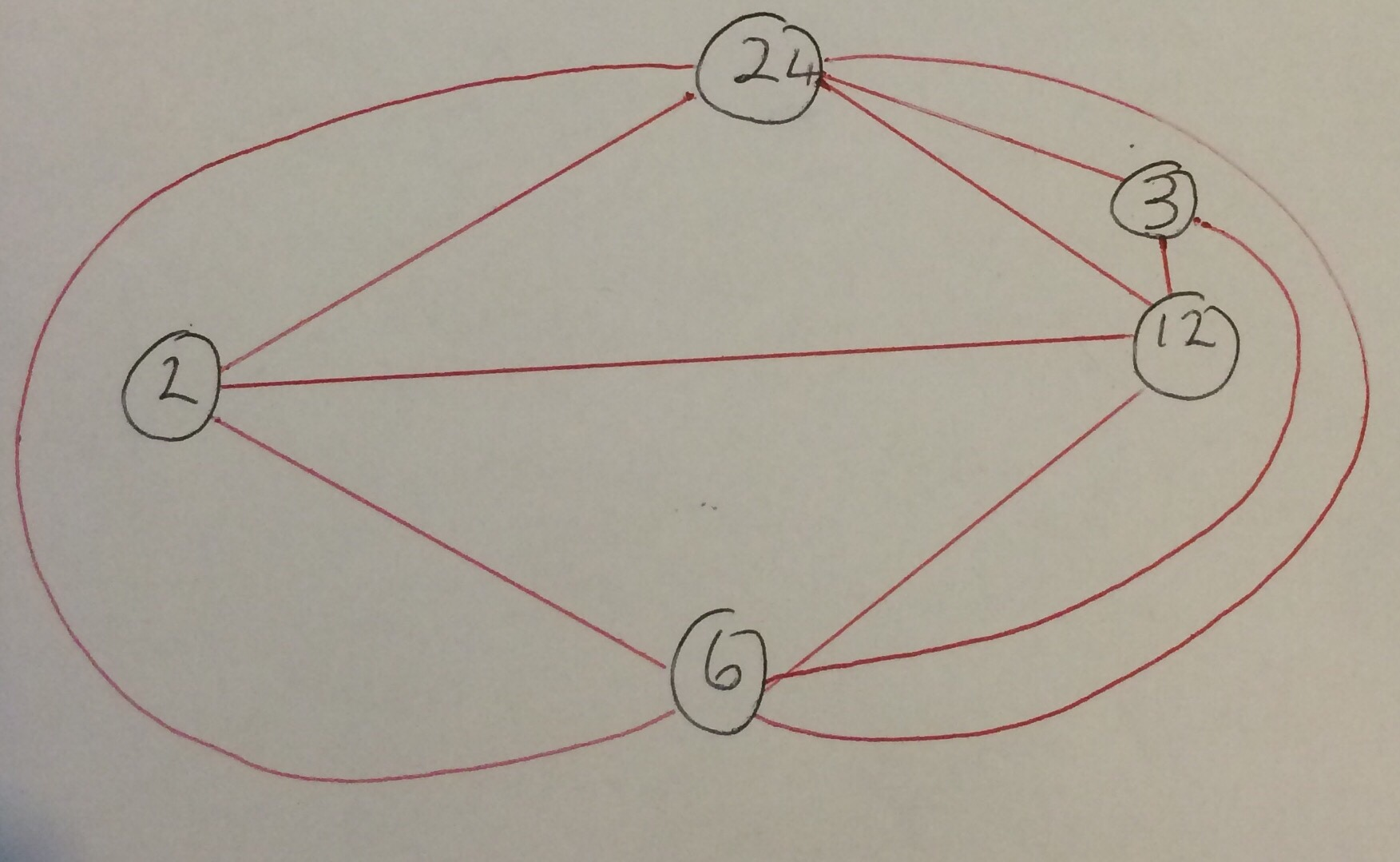However, looking at the graph for the numbers 2 to 23 (which is possible):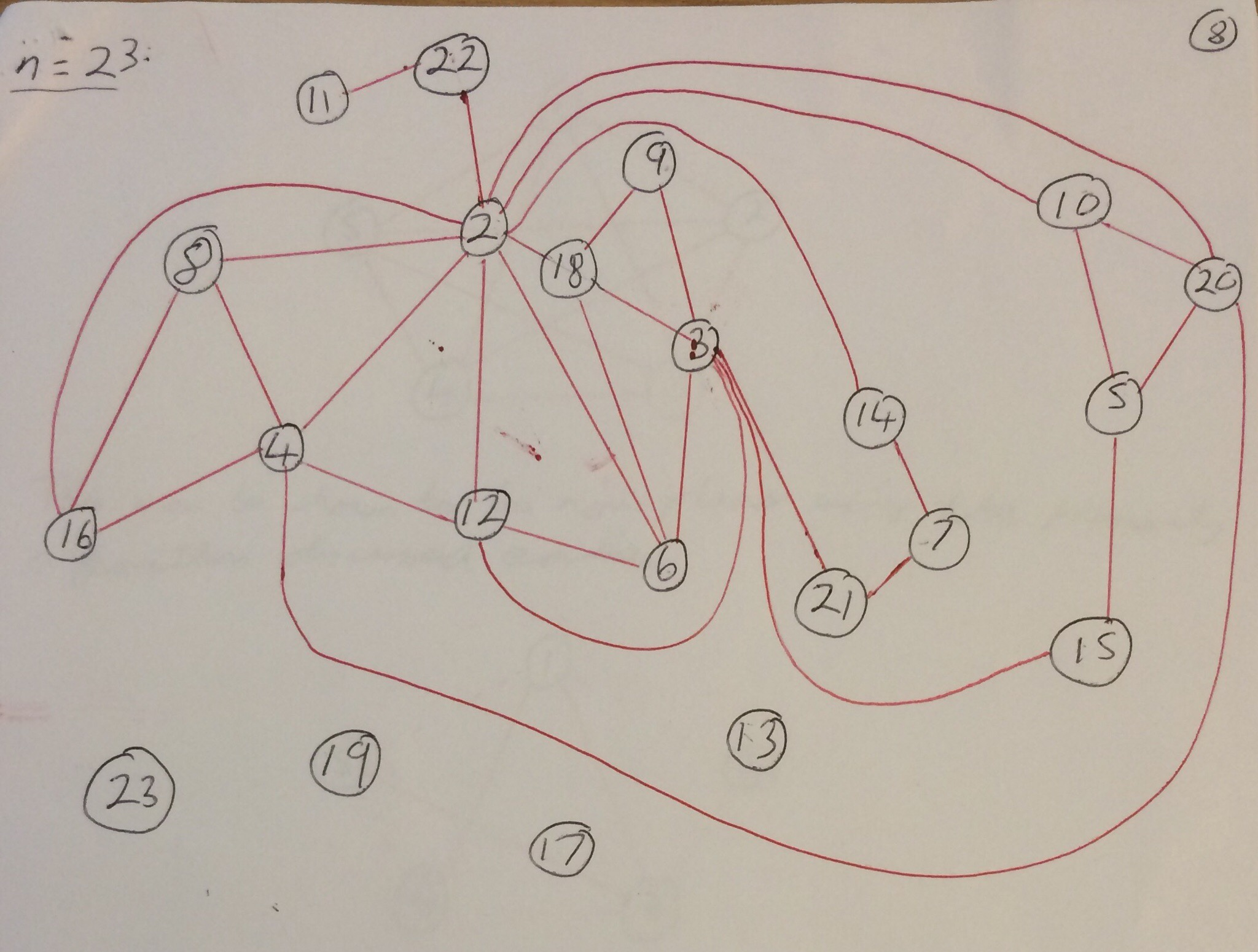we can see numerous indirect paths joining 2 to 3, for example 2-18-3, 2-14-7-21-3, 2-6-3, 2-10-20-5-15-3 and 2-12-6-3. It can be seen from our diagram that we cannot add any of these paths to our diagram without introducing a crossing.

Another way to show the non-planirity of the graph containing all the integers 2 to 24 is to relate it to the famous “3 utilities problem”.

I enjoyed this problem, but I didn’t like the fact that you also need to draw the graph for the numbers 2 to 23 to show that 24 is the smallest integer that won’t work.